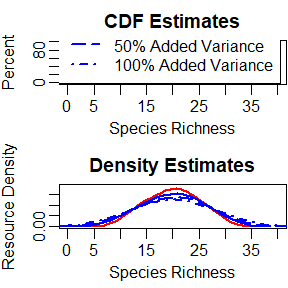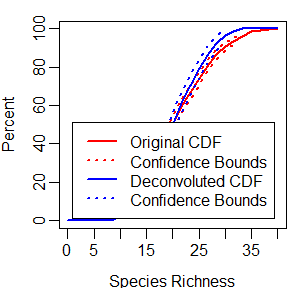# CDF Deconvolution

## Introduction

This document presents deconvolution of a cumulative distribution function (CDF). Convolution is a term that refers to a distribution that is a mixture of two or more component distributions. Specifically, we are interested in the situation where the CDF is a mixture of the distribution for a variable of interest and measurement error. The presence of measurement error will cause the CDF to occur across a wider range of variable values, which will lead to biased estimation of the CDF. Deconvolution is the name of the procedure for removing the measurement error from the CDF. In order to illustrate the deconvolution process, simulated data is used rather than data generated by a probability survey. For additional discussion of measurement error and deconvolution see Kincaid and Olsen (Kincaid and Olsen 2012).

## Preliminaries

The initial step is to use the library function to load the spsurvey package. After the package is loaded, a message is printed to the R console indicating that the spsurvey package was loaded successfully.

Load the spsurvey package:

library(spsurvey)

## Load the Simulated Variables Data Set

A data set was created that contains simulated species richness variables. The initial variable was created using the Normal distribution with mean 20 and standard deviation 5. Addition variables were created by adding 25%, 50%, and 100% measurement error variance to the original variable, where the percent values refer to the variance of the original variable. The data file also includes x-coordinates and y-coordinates, which were simulated using the Uniform distribution with a range from zero to one. The data set contains 500 records.

The next step is to load the data set. The data function is used to load the data set and assign it to a data frame named decon_data. The nrow function is used to determine the number of rows in the decon_data data frame, and the resulting value is assigned to an object named nr. Finally, the initial six lines and the final six lines in the decon_data data frame are printed using the head and tail functions, respectively.

Load the simulated variables data set:

data(decon_data)
nr <- nrow(decon_data)

Display the initial six lines in the data file:

head(decon_data)
#>      xcoord    ycoord  Richness Richness_25 Richness_50 Richness_100
#> 1 0.2156870 0.1040707  9.195568   10.413331    2.291196     2.819672
#> 2 0.7253217 0.4319726  8.853398    9.521474    4.521184     8.183711
#> 3 0.3200359 0.4419541  8.631306    3.747440    4.815115     5.909885
#> 4 0.9686209 0.8958557 10.361718   10.973729    5.192450     9.874936
#> 5 0.0974488 0.1890712 12.424943   10.121154    5.235458    14.121246
#> 6 0.8153121 0.6643893  9.635846    9.413341    5.872094    16.581228

Display the final six lines in the data file:

tail(decon_data)
#>        xcoord     ycoord Richness Richness_25 Richness_50 Richness_100
#> 495 0.7431377 0.68816008 28.40369    29.78458    33.61083     27.16529
#> 496 0.8238306 0.92598006 30.17266    31.48048    33.89747     34.05622
#> 497 0.8884304 0.07949327 26.96940    27.06877    34.58659     33.63208
#> 498 0.5394230 0.60650557 29.80406    26.15533    34.93280     22.27781
#> 499 0.4200749 0.28636323 28.68552    29.03119    36.22896     32.96995
#> 500 0.5356669 0.64663409 30.65390    30.58069    37.46364     30.13545

## Illustration of Extraneous Variance

Measurement error is an example of extraneous variance, additional variance that is convoluted with the population frequency distribution for a variable of interest. The impact of extraneous variance on the CDF will be investigated in this section. As an initial step, the summary function is used to summarize the data structure of the species richness values for the original variable.

cat("\nSummarize the data structure of the species richness variable:\n")
#>
#> Summarize the data structure of the species richness variable:
summary(decon_data$Richness) #> Min. 1st Qu. Median Mean 3rd Qu. Max. #> 8.631 15.958 20.093 19.999 23.611 30.658 Note that the species richness values range between approximately 8 and 31. By way of comparison, consider the range of value for the original variable plus 100% additional measurement error. cat("\nSummarize the data structure of the species richness variable plus \n100% measurrement error:\n") #> #> Summarize the data structure of the species richness variable plus #> 100% measurrement error: summary(decon_data$Richness_100)
#>    Min. 1st Qu.  Median    Mean 3rd Qu.    Max.
#>   1.274  15.312  20.026  20.150  25.078  39.652

The range of species richness values now has increased to 1 to 40, which reflects the bias that is introduced into the CDF. To illustrate the bias induced by extraneous variance, CDFs for the species richness variables will be graphed. The first step is to assign a vector of values at which the CDFs will be calculated. The sequence (seq) function is used to create the set of values using the range 0 to 40. Output from the seq function is assigned to an object named cdfvals.

cdfvals <- seq(0,40,length=25)

The next step is to calculate a CDF estimate for the original species richness variable. The cdf.est function in spsurvey will be used to calculate the estimate. Since values for the species richness variables were not selected in a probability survey, the survey design weights argument (wgt) for the function is assigned a vector of ones using the repeat (rep) function. Recall that the object named nr contains the number of rows in the decon_data data frame.

CDF_org <- cdf.est(z=decon_data$Richness, wgt=rep(1, nr), x=decon_data$xcoord,
y=decon_data$ycoord, cdfval=cdfvals) Similarly, the cdf.est function will be used to calculate CDF estimates for the species richness variables that include measurement error. # Calculate a CDF estimate for the variable plus 25% extraneous variance CDF_25 <- cdf.est(z=decon_data$Richness_25,
wgt=rep(1, nrow(decon_data)),
x=decon_data$xcoord, y=decon_data$ycoord,
cdfval=cdfvals)

# Calculate a CDF estimate for the variable plus 50% extraneous variance
CDF_50 <- cdf.est(z=decon_data$Richness_50, wgt=rep(1, nrow(decon_data)), x=decon_data$xcoord,
y=decon_data$ycoord, cdfval=cdfvals) # Calculate a CDF estimate for the variable plus 100% extraneous variance CDF_100 <- cdf.est(z=decon_data$Richness_100,
wgt=rep(1, nrow(decon_data)),
x=decon_data$xcoord, y=decon_data$ycoord,
cdfval=cdfvals)

Density estimates facilitate visualizing the increased variance induced in a CDF by measurement error. The ash1.wgt function in spsurvey, which implements the average shifted histogram algorithm (Scott 1985), will be used to calculate density estimates for each of the species richness variables.

Density_org <- ash1.wgt(decon_data$Richness, nbin=25) Density_25 <- ash1.wgt(decon_data$Richness_25, nbin=25)
Density_50 <- ash1.wgt(decon_data$Richness_50, nbin=25) Density_100 <- ash1.wgt(decon_data$Richness_100, nbin=25)

CDF and density estimates are displayed in the figure below. Estimates for the original species richness variable are displayed using red. Estimates for variables containing measurement error are displayed using blue.

op <- par(mfrow=c(2,1), mgp=c(2.2,0.6,0), mar=c(3,3,2,0)+0.1)

plot(CDF_org$CDF$Value, CDF_org$CDF$Estimate.P, type="l", lwd=2, col="red",
xlim=c(0, 40), ylim=c(0, 100), xaxt="n", xlab="", ylab="Percent")
title(main="CDF Estimates")
axis(side=1, at=c(0, 5, 10, 15, 20, 25, 30, 35, 40),
labels=c("0", "5", "10", "15", "20", "25", "30", "35", "40"))
mtext("Species Richness", side=1, line=1.75, cex=par("cex"))
lines(CDF_25$CDF$Value, CDF_25$CDF$Estimate.P, lwd=2, col="blue")
lines(CDF_50$CDF$Value, CDF_50$CDF$Estimate.P, lty=5, lwd=2.5,
col="blue")
lines(CDF_100$CDF$Value, CDF_100$CDF$Estimate.P, lty=4, lwd=2.5,
col="blue")
legend(x="bottomright", inset=0.025, legend=c("Original Variable",
"100% Added Variance"), lty=c(1,1,5,4), lwd=c(2,2,2.5, 2.5),
bty="o", cex=1, col=c("red", "blue", "blue", "blue"))

plot(Density_org$x, Density_org$y, type="l", lwd=2, col="red",
xlim=c(0, 40), ylim=c(0, 0.075), xaxt="n", xlab="",
ylab="Resource Density")
title(main="Density Estimates")
axis(side=1, at=c(0, 5, 10, 15, 20, 25, 30, 35, 40),
labels=c("0", "5", "10", "15", "20", "25", "30", "35", "40"))
mtext("Species Richness", side=1, line=1.75, cex=par("cex"))
lines(Density_25$x, Density_25$y, lwd=2, col="blue")
lines(Density_50$x, Density_50$y, lty=5, lwd=2.5, col="blue")
lines(Density_100$x, Density_100$y, lty=4, lwd=2.5, col="blue")CDF and Density Estimates for Species Richness Variables.


par(op)

## Deconvolution

Deconvolution will be demonstrated using the species richness variable that includes 100% additional measurement error variance. The decon.est function in spsurvey will be used to calculate a deconvoluted CDF estimate, which uses an algorithm based on the procedure developed by Stefansky and Bay (Stefansky and Bay 1996). Argument sigma for that function specifies the extraneous variance. For an actual survey design, a value for sigma would be estimated from the survey data or obtained from some other source. Since we are using simulated data, a direct estimate of sigma is available. Specifically, the variance estimation function (var) will be used to estimate the additional variance for the species richness variable with added measurement error, and the result will be assigned to an object named extvar.

extvar <- var(decon_data$Richness_100) - var(decon_data$Richness)
CDF_decon <- cdf.decon(z=decon_data$Richness_100, wgt=rep(1,nr), sigma=extvar, x=decon_data$xcoord,
y=decon_data$ycoord, cdfval=cdfvals) The original CDF and deconvoluted CDF estimates for the species richness variable that includes 100% additional measurement error variance are displayed in the figure below. The CDF estimate and confidence bounds for the original variable are displayed in red. The deconvoluted CDF estimate and confidence bound are displayed in blue. One consequence of deconvolution is increased confidence bound width, which can be observed in the CDFs in the figure below. For example, for a species richness value of 25, the confidence bounds for the original variable are 78.7% to 84.5%, which is a width of 5.8%. For the deconvoluted CDF, the confidence bounds are 74.2% to 83.9%, which is a width of 9.7%. op <- par(mgp=c(2.2,0.6,0), mar=c(3,3,1,0)+0.1) plot(CDF_100$CDF$Value, CDF_100$CDF$Estimate.P, type="l", lwd=2, col="red", xlim=c(0, 40), xaxt="n", xlab="Species Richness", ylab="Percent") axis(side=1, at=c(0, 5, 10, 15, 20, 25, 30, 35, 40), labels=c("0", "5", "10", "15", "20", "25", "30", "35", "40")) confcut <- 5 pctval <- c(confcut, 100-confcut) tvalue <- CDF_100$CDF$Estimate.P >= pctval & CDF_100$CDF$Estimate.P <= pctval x <- interp.cdf(pctval, CDF_100$CDF$Estimate.P, CDF_100$CDF$Value) ylow <- interp.cdf(pctval, CDF_100$CDF$Estimate.P, CDF_100$CDF$LCB95Pct.P) yhi <- interp.cdf(pctval, CDF_100$CDF$Estimate.P, CDF_100$CDF$UCB95Pct.P) value <- c(x, CDF_100$CDF$Value[tvalue], x) lower <- c(ylow, CDF_100$CDF$LCB95Pct.P[tvalue], ylow) upper <- c(yhi, CDF_100$CDF$UCB95Pct.P[tvalue], yhi) lines(value, lower, lty=3, lwd=2.5, col="red") lines(value, upper, lty=3, lwd=2.5, col="red") lines(CDF_decon$CDF$Value, CDF_decon$CDF$Estimate.P, lwd=2, col="blue") tvalue <- CDF_decon$CDF$Estimate.P >= pctval & CDF_decon$CDF$Estimate.P <= pctval x <- interp.cdf(pctval, CDF_decon$CDF$Estimate.P, CDF_decon$CDF$Value) ylow <- interp.cdf(pctval, CDF_decon$CDF$Estimate.P, CDF_decon$CDF$LCB95Pct.P) yhi <- interp.cdf(pctval, CDF_decon$CDF$Estimate.P, CDF_decon$CDF$UCB95Pct.P) value <- c(x, CDF_decon$CDF$Value[tvalue], x) lower <- c(ylow, CDF_decon$CDF$LCB95Pct.P[tvalue], ylow) upper <- c(yhi, CDF_decon$CDF\$UCB95Pct.P[tvalue], yhi)
lines(value, lower, lty=3, lwd=2.5, col="blue")
lines(value, upper, lty=3, lwd=2.5, col="blue")
legend(x="bottomright", inset=0.05, legend=c("Original CDF",
"Confidence Bounds", "Deconvoluted CDF", "Confidence Bounds"),
lty=c(1,3,1,3), lwd=c(2,2.5,2,2.5), bty="o", cex=1,
col=c("red", "red", "blue", "blue"))Original and Deconvoluted CDF Estimates for a Species Richness Variable with Added Measurement Error.


par(op)